# A Visual Exploration of DeepCluster

Many self-supervised methods use pretext tasks to generate surrogate labels and formulate an unsupervised learning problem as a supervised one. Some examples include rotation prediction, image colorization, jigsaw puzzles etc. However, such pretext tasks are domain-dependent and require expertise to design them.

DeepCluster is a self-supervised method proposed by Caron et al. of Facebook AI Research that brings a different approach. This method doesn’t require domain-specific knowledge and can be used to learn deep representations for scenarios where annotated data is scarce.

## DeepCluster

DeepCluster combines two pieces: unsupervised clustering and deep neural networks. It proposes an end-to-end method to jointly learn parameters of a deep neural network and the cluster assignments of its representations. The features are generated and clustered iteratively to get both a trained model and labels as output artifacts.

## Deep Cluster Pipeline

Let’s now understand how the deep cluster pipeline works with an interactive diagram.Synopsis:
As seen in the figure above, unlabeled images are taken and augmentations are applied to them. Then, an ConvNet architecture such as AlexNet or VGG-16 is used as the feature extractor. Initially, the ConvNet is initialized with randomly weights and we take the feature vector from layer before the final classification head. Then, PCA is used to reduce the dimension of the feature vector along with whitening and L2 normalization. Finally, the processed features are passed to K-means to get cluster assignment for each image.

These cluster assignments are used as the pseudo-labels and the ConvNet is trained to predict these clusters. Cross-entropy loss is used to gauge the performance of the model. The model is trained for 100 epochs with the clustering step occurring once per epoch. Finally, we can take the representations learned and use it for downstream tasks.

## Step by Step Example

Let’s see how DeepCluster is applied in practice with a step by step example of the whole pipeline from the input data to the output labels:

1. Training Data
We take unlabeled images from the ImageNet dataset which consist of 1.3 million images uniformly distributed into 1000 classes. These images are prepared in mini-batches of 256.The training set of N images can be denoted mathematically by:

$X = \{ x_{1}, x_{2}, ..., x_{N} \}$

2. Data Augmentation

Transformations are applied to the images so that the features learned is invariant to augmentations. Two different augmentations are done, one when training model to learn representations and one when sending the image representations to the clustering algorithm:

Case 1: Transformation when doing clustering

When model representations are to be sent for clustering, random augmentations are not used. The image is simply resized to 256*256 and the center crop is applied to get 224*224 image. Then normalization is applied.In PyTorch, this can be implemented as:

from PIL import Image
import torchvision.transforms as transforms

im = Image.open('dog.png')
t = transforms.Compose([transforms.Resize(256),
transforms.CenterCrop(224),
transforms.ToTensor(),
transforms.Normalize(mean=[0.485, 0.456, 0.406],
std=[0.229, 0.224, 0.225])])
aug_im = t(im)


Case 2: Transformation when training model

When the model is trained on image and labels, then we use random augmentations. The image is cropped to a random size and aspect ratio and then resized to 224*224. Then, the image is horizontally flipped with a 50% chance. Finally, we normalize the image with ImageNet mean and std.In PyTorch, this can be implemented as:

from PIL import Image
import torchvision.transforms as transforms

im = Image.open('dog.png')
t = transforms.Compose([transforms.RandomResizedCrop(224),
transforms.RandomHorizontalFlip(),
transforms.ToTensor(),
transforms.Normalize(mean=[0.485, 0.456, 0.406],
std=[0.229, 0.224, 0.225])])
aug_im = t(im)


Sobel Transformation

Once we get the normalized image, we convert it into grayscale. Then, we increase the local contrast of the image using the Sobel filters.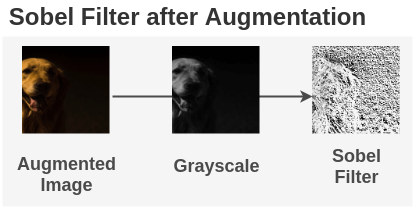Below is a simplified snippet adapted from the author’s implementation here. We can apply it on the augmented image aug_im we got above.

import torch
import torch.nn as nn

# Fill kernel of Conv2d layer with grayscale kernel
grayscale = nn.Conv2d(3, 1, kernel_size=1, stride=1, padding=0)
grayscale.weight.data.fill_(1.0 / 3.0)
grayscale.bias.data.zero_()

# Fill kernel of Conv2d layer with sobel kernels
sobel = nn.Conv2d(1, 2, kernel_size=3, stride=1, padding=1)
sobel.weight.data[0, 0].copy_(
torch.FloatTensor([[1, 0, -1],
[2, 0, -2],
[1, 0, -1]])
)
sobel.weight.data[1, 0].copy_(
torch.FloatTensor([[1, 2, 1],
[0, 0, 0],
[-1, -2, -1]])
)
sobel.bias.data.zero_()

# Combine the two
combined = nn.Sequential(grayscale, sobel)

# Apply
batch_image = aug_im.unsqueeze(dim=0)
sobel_im = combined(batch_image)


3. Decide Number of Clusters(Classes)

To perform clustering, we need to decide the number of clusters. This will be the number of classes the model will be trained on.By default, ImageNet has 1000 classes, but the paper uses 10,000 clusters as this gives more fine-grained grouping of the unlabeled images. For example, if you previously had a grouping of cats and dogs and you increase clusters, then groupings of breeds of the cat and dog could be created.

4. Model Architecture

The paper primarily uses AlexNet architecture consisting of 5 convolutional layers and 3 fully connected layers. The Local Response Normalization layers are removed and Batch Normalization is applied instead. Dropout is also added. The filter size used is from 2012 competition: 96, 256, 384, 384, 256.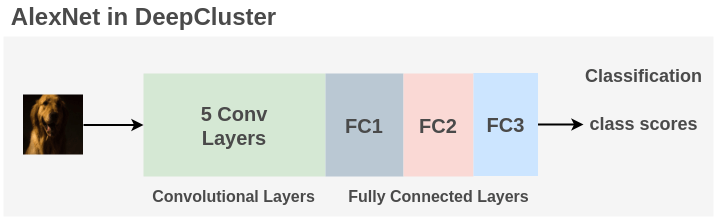Alternatively, the paper has also tried replacing AlexNet by VGG-16 with batch normalization to see impact on performance.

5. Generating the initial labels

To generating initial labels for the model to train on, we initialize AlexNet with random weights and the last fully connected layer FC3 removed. We perform a forward pass on the model on images and take the feature vector coming from the second fully connected layer FC2 of the model on an image. This feature vector has a dimension of 4096.This process is repeated for all images in the batch for the whole dataset. Thus, if we have N total images, we will have an image-feature matrix of [N, 4096].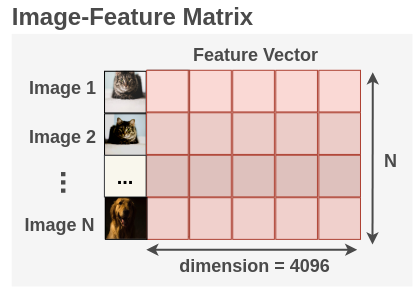6. Clustering

Before performing clustering, dimensionality reduction is applied to the image-feature matrix.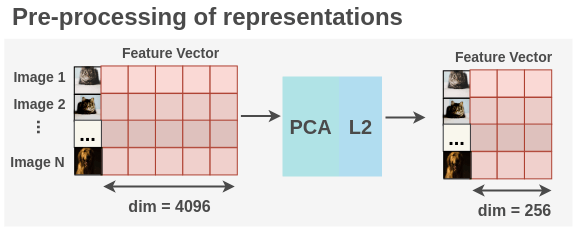For dimensionality reduction, Principal Component Analysis(PCA) is applied to the features to reduce them from 4096 dimensions to 256 dimensions. The values are also whitened.
The paper uses the faiss library to perform this at scale. Faiss provides an efficient implementation of PCA which can be applied for some image-feature matrix x as:

import faiss

# Apply PCA with whitening
mat = faiss.PCAMatrix(d_in=4096, d_out=256, eigen_power=-0.5)
mat.train(x)
x_pca = mat.apply_py(x)


Then, L2 normalization is applied to the values we get after PCA.

import numpy as np

norm = np.linalg.norm(x_pca, axis=1)
x_l2 = x_pca / norm[:, np.newaxis]


Thus, we finally get a matrix of (N, 256) for total N images. Now, K-means clustering is applied to the pre-processed features to get images and their corresponding clusters. These clusters will act as the pseudo-labels on which the model will be trained.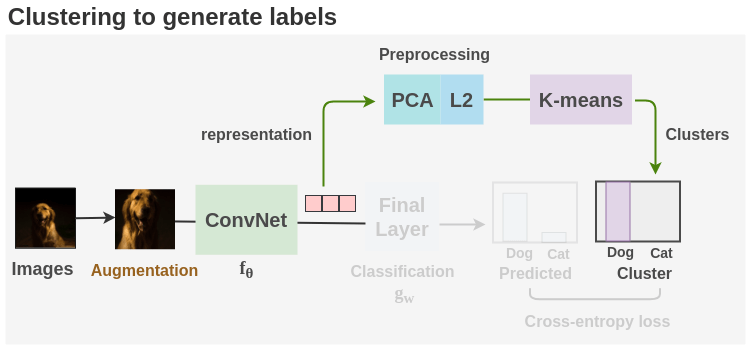The paper use Johnson’s implementation of K-means from the paper “Billion-scale similarity search with GPUs”. It is available in the faiss library. Since clustering has to be run on all the images, it takes one-third of the total training time.

After clustering is done, new batches of images are created such that images from each cluster has an equal chance of being included. Random augmentations are applied to these images.

7. Representation Learning

Once we have the images and clusters, we train our ConvNet model like regular supervised learning. We use a batch size of 256 and use cross-entropy loss to compare model predictions to the ground truth cluster label. The model learns useful representations.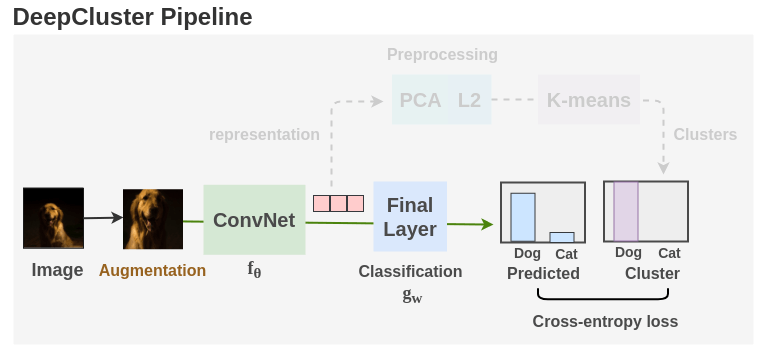8. Switching between model training and clustering

The model is trained for 500 epochs. The clustering step is run once at the start of each epoch to generate pseudo-labels for the whole dataset. Then, the regular training of ConvNet using cross-entropy loss is continued for all the batches. The paper uses SGD optimizer with momentum of 0.9, learning rate of 0.05 and weight decay of $10^{-5}$. They trained it on Pascal P100 GPU.

## Code Implementation of DeepCluster

The official implementation of Deep Cluster in PyTorch by the paper authors is available on GitHub. They also provide pretrained weights for AlexNet and Resnet-50 architectures.

## Citation Info (BibTex)

If you found this blog post useful, please consider citing it as:

@misc{chaudhary2020DeepCluster,
title   = {A Visual Exploration of DeepCluster},
author  = {Amit Chaudhary},
year    = 2020,
note    = {\url{https://amitness.com/2020/04/illustrated-deepcluster}}
}